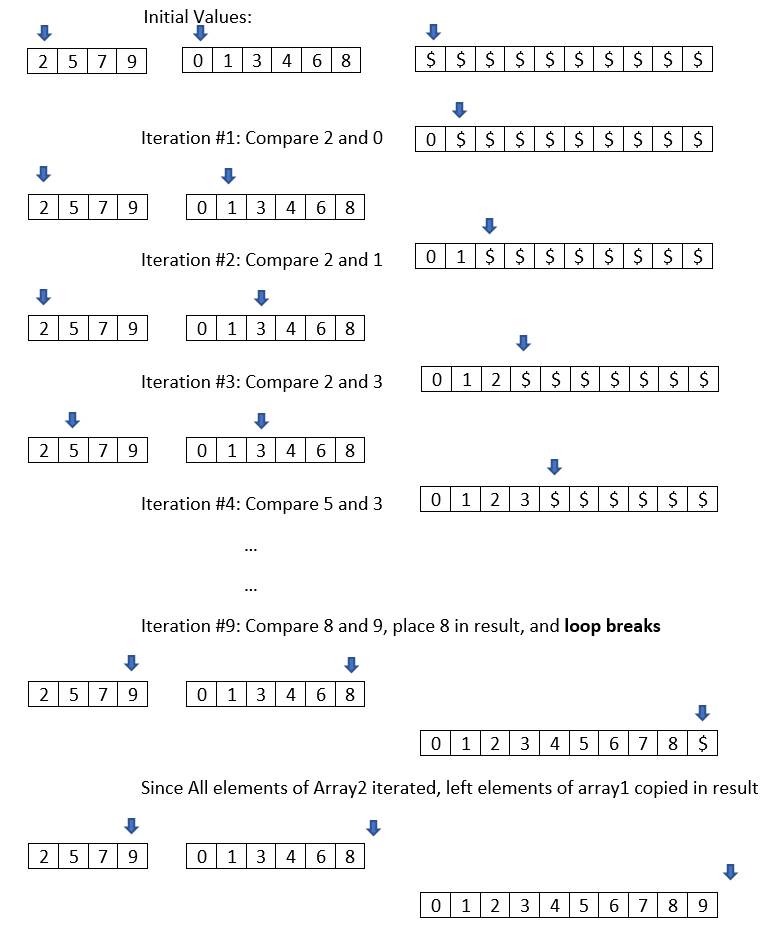Merge two sorted arrays in one in O(n) time, merge two sorted arrays using merge sort technique

 Category: Data Structure And Algorithms Tags:Merge two sorted array code files

You would have been asked in interviews how to merge two sorted arrays in O(n) time complexity. It’s quite simple if you know Merge Sort. Merge Sort works on divide and conquer technique, Merge Sort divides array in smallest chunks and starts merging them. While merging, it takes one element from each of the array, compare it and place smaller one on output array. You can read about merge sort here.

I’m going to use same technique to merge two sorted arrays. Since we already have sorted arrays we no need to divide them but we just need to merge them. Suppose we have two sorted arrays array1, array2 and one result array of size (array1+ array2). We can merge them illustrated in figure below:Fig 1: Merging two sorted arrays

Implementation

See below code and go through the code comments:

static void Main(string[] args)
{
int[] array1 = new int[] { 2, 5, 7, 9 };
int[] array2 = new int[] { 0, 1, 3, 4, 6, 8 };
int[] arrayResult = new int[array1.Length + array2.Length];

//index for result
int n = 0;
//index for array1
int i = 0;
//index for array2
int j = 0;

//until any one of arrays all elements are traversed
while (i < array1.Length && j < array2.Length)
{
//comparing items of array1 and array2
if (array1[i] < array2[j])
{
//inserting array1 item since it is lower
arrayResult[n] = array1[i];
//incrementing i, because array1's item is traversed
i++;
}
else
{
arrayResult[n] = array2[j];
j++;
}
//incrementing since one item has been inserted to result array
n++;
}
//if any elements are left in array1, simply inserting all to result
while (i < array1.Length)
{
arrayResult[n] = array1[i];
i++;
n++;
}
//if any elements are left in array2, simply inserting all to result
while (j < array2.Length)
{
arrayResult[n] = array2[j];
j++;
n++;
}
//printing result array
foreach (int ele in arrayResult)
Console.WriteLine(ele);
}

Output

0

1

2

3

4

5

6

7

8

9

In above code we have iterated all elements of array1 and array2 once, so iterations would be (i + j). Complexity will be O(i + j) which is linear time, simply we can call it O(n) where n = (length of array1 + length of array2) or (i + j).

 Like0 PeopleNikhil Joshi Ceo & Founder at Dotnetlovers Atricles: 133 Questions: 9 Given Best Solutions: 9 *

Login via:x